#### Vol. 15, No. 3, 1965

 Download this articleFor screen For printingRecent Issues Vol. 325: 1  2 Vol. 324: 1  2 Vol. 323: 1  2 Vol. 322: 1  2 Vol. 321: 1  2 Vol. 320: 1  2 Vol. 319: 1  2 Vol. 318: 1  2Online Archive Volume: Issue:The Journal Subscriptions Editorial Board Officers Contacts Submission Guidelines Submission Form Policies for Authors ISSN: 1945-5844 (e-only) ISSN: 0030-8730 (print) Special Issues Author Index To Appear Other MSP Journals
L2 expansions in terms of generalized heat polynomials and of their Appell transforms

### Deborah Tepper Haimo

Vol. 15 (1965), No. 3, 865–875
##### Abstract

The object of this paper is to characterize functions which have L2 expansions in terms of polynomial solutions Pn,ν(x,t) of the generalized heat equation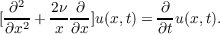(*)

and in terms of the Appell transforms Wn,ν(x,t) of the Pn,ν(x,t). H denotes the C2 class of functions u(x,t) which, for a < t < b, satisfy (*) and for which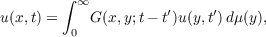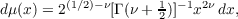for all t, t, a < t< t < b, the integral converging absolutely, where G(x,y;t) is the source solution of (*). The principal results are the following:

Theorem. Let u(x,t) H,  σ t < 0, and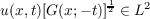for each fixed t σ t < 0, 0 x < . Then, for σ t < 0,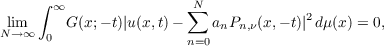and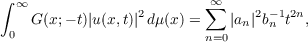where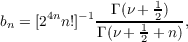and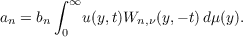Theorem. If u(x,t) H, 0 < t σ, and if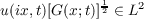for each fixed t, 0 < t σ, 0 x < , then, for 0 < t σ,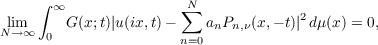and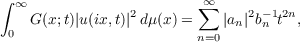where bn is given above and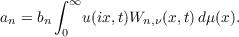Theorem. If u(x,t) H, 0 < σ t, and if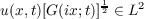for each fixed t, 0 < σ t, 0 x < , then, for 0 < σ t,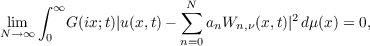and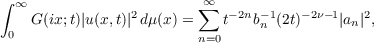where bn is given above and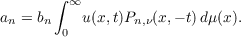Primary: 44.30
Secondary: 33.00
##### Milestones